# UWB Channel Models

This example shows how to implement UWB channel models for a variety of propagation environments. The implementation is based on recommendations from the channel modeling subgroup of IEEE® 802.15.4a™ . You can reuse these UWB channel models for the IEEE 802.15.4z amendment , which enhances the HRP PHY introduced by IEEE 802.15.4a.

### Overview

The recommended UWB channel models comprise the following parts:

1. Application of distance- and frequency-dependent path loss

2. A modified Saleh-Valenzuela model , which contains multipath components that are grouped in distinct clusters

3. Determination of path amplitudes with distinct Nakagami distributions (small-scale fading)

4. Environment-specific parameterization of the above steps

First, this example sequentially illustrates each component of the UWB channel model. Next, in the end of this example, an aggregate `uwbChannel` object is used to implement all aspects of the UWB channel model with a single command.

### Environment Parameterization

The IEEE 802.15.4a channel modeling subgroup recommends multiple UWB models for the 2 to 10 GHz range, which cover a wide range of environments:

• Residential (indoor)

• Indoor office

• Outdoor (suburban-like)

• Open outdoor (agricultural)

• Industrial (indoor)

The subgroup recommends two distinct models for each environment type (except for open outdoor), depending on the presence or absence of a line-of-sight (LOS) component. Thus, this example implements a total of nine combinations (type x LOS) for the 2 to 10 GHz range.

To create a channel-model parameterization for a specific scenario, construct a `uwbChannelModel` object accordingly.

```environmentType =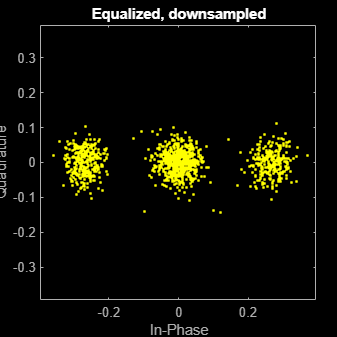"Indoor office"; LOS =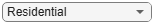true; env = uwbChannelConfig(environmentType, LOS)```
```env = uwbChannelConfig with properties: Type: 'Indoor office' HasLOS: 1 ReferencePathLoss: 35.4000 PathLossExponent: 1.6300 ShadowingDeviation: 1.9000 AntennaLoss: 3 FrequencyExponent: 0.0300 AverageNumClusters: 5.4000 ClusterArrivalRate: 0.0160 PathArrivalRate1: 0.1900 PathArrivalRate2: 2.9700 MixtureProbability: 0.0184 ClusterEnergyDecayConstant: 14.6000 PathDecaySlope: 0 PathDecayOffset: 6.4000 NakagamiMeanOffset: 0.4200 NakagamiMeanSlope: 0 NakagamiDeviationOffset: 0.3100 NakagamiDeviationSlope: 0 ```

You can create custom environment scenarios by modifying the configuration presets above.

### Propagation and Path Loss

The received signal experiences path loss and suffers a reduction in power. The model in  recommends that path loss `PL` depends on the distance `d` between the transmitter and the receiver, and also that path loss manifests nonuniformly for the different frequencies `f` of the received signal.

`$\mathrm{PL}{\left(\mathit{f},\mathit{d}\right)=\mathrm{PL}\left(\mathit{f}\right)*\mathrm{PL}\left(\mathit{d}\right)}_{}$`

Distance and frequency affect path loss in a manner specific to each environment type.

#### Transmitted Signal

First, create a transmitted signal that experiences path loss. Here, an IEEE 802.15.4a/z signal is created, but the example can also be used with signals of different types.

```Ptx =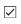1; % Transmit power, in Watts % Create IEEE 802.15.4a/z signal: psduLen = 10; % bytes psdu = randi([0, 1], psduLen*8, 1); cfgBPRF = lrwpanHRPConfig(Mode='BPRF', ... STSPacketConfiguration=0, ... PHRDataRate=6.81, ... % PHR at 6.81 Mbps (BPRF payload always at 6.81 Mbps) CodeIndex=9, ... % One of the 127-symbols long SYNC codes PreambleDuration=16, ... PSDULength=psduLen); waveBPRF = lrwpanWaveformGenerator(psdu, cfgBPRF); waveBPRF = waveBPRF*sqrt(Ptx)/(sqrt(mean(waveBPRF.^2)));```

#### Distance Dependence

Establish the path loss dependence on distance with the classic log-distance propagation model. A path loss exponent $\mathit{n}$ (`PathLossExponent` in `uwbChannelConfig`, specific to environment) determines how quickly power dissipates as a function of distance `d`. A zero-mean Gaussian random variable models shadowing (as seen in large-scale fading, denoted by `S`).

`$P{L}^{\mathrm{dB}}\left(\mathit{d}\right)=\mathit{P}{\mathit{L}}_{0}+10*n*{\mathrm{log}}_{10}\left(\frac{\mathit{d}}{{\mathit{d}}_{0}}\right)+\mathit{S}$`

`PL0` is the reference path loss at the reference distance `d0` (1 m).

```d =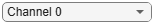10; % distance between transmitter and receiver, in meters rxSignal = helperDistancePathLoss(waveBPRF, env.ReferencePathLoss, d, env.PathLossExponent, env.ShadowingDeviation);```

#### Frequency Dependence

Model frequency dependence by amplifying frequencies smaller than the center frequency ${\mathit{f}}_{\mathit{c}}$ and reducing the power of higher frequencies. The power differences within the same bandwidth decrease with the center frequency ${\mathit{f}}_{\mathit{c}}$. A path loss exponent $\kappa$ (`FrequencyExponent` in `uwbChannelConfig`, specific to environment) determines how quickly power is lost as a function of frequency.

`$\mathrm{PL}\left(\mathit{f}\right)={\left(\frac{\mathit{f}}{{\mathit{f}}_{\mathit{c}}}\right)}^{2\kappa +1}$`

To apply frequency-dependent path loss, select an IEEE 802.15.4a/z channel number, to determine the center frequency ${\mathit{f}}_{\mathit{c}}\text{\hspace{0.17em}}$and the channel bandwidth (see Table 15-11 in ).

```channelSpec =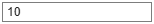[0 499.2e6 499.2e6]; channelNum = channelSpec(1); Fc = channelSpec(2); bw = channelSpec(3); fprintf('Channel #%d: Center frequency = %.1f MHz, Bandwidth = %.1f MHz.\n', channelNum, Fc/1e6, bw/1e6);```
```Channel #0: Center frequency = 499.2 MHz, Bandwidth = 499.2 MHz. ```

Next, create an arbitrary-magnitude filter to enable the frequency-dependent profile described above.

`rxSignal = helperFrequencyPathLoss(rxSignal, Fc, bw, env.FrequencyExponent);`

#### Antenna Effects

Finally, the recommended model describes two antenna-related effects. First, the presence of people close to antennas is modeled as a static "antenna attenuation factor". Second, the environment parameterization contains an "antenna loss" factor (`AntennaLoss` in `uwbChannelConfig`), which presumably models the overall effect of both the transmit and receive antennas.

```rxSignal = rxSignal/2; % presence of people ("antenna attenuation factor"), see Section II.B.4 in  rxSignal = rxSignal * 10^(-env.AntennaLoss/20); % apply antenna loss (convert from dB to V)```

### Power Delay Profile

The recommended UWB channel model is based on the Saleh-Valenzuela model  and contains multipath components that are grouped into `L` clusters.

`$\mathit{h}\left(\mathit{t}\right)=\sum _{\mathit{l}=0}^{\mathit{L}}\sum _{\mathit{k}=0}^{\mathit{K}}{\mathit{a}}_{\mathit{k},\mathit{l}}*{\mathit{e}}^{\mathit{j}{\phi }_{\mathit{k},\mathit{l}}}\delta \left(\mathit{t}-{\mathit{T}}_{\mathit{l}}-{\tau }_{\mathit{k},\mathit{l}}\right)$`

The amplitude, phase and delay of the `kth` path in the `lth` cluster are denoted by ${\mathit{a}}_{\mathit{k},\mathit{l}}$, ${\phi }_{\mathit{k},\mathit{l}}$ and ${\tau }_{\mathit{k},\mathit{l}}$ respectively.

#### Clusterization

• Number of clusters: The number of clusters (`L`) follows a Poisson distribution, and its average number is environment-specific.

• Cluster arrival times: Each of these clusters has an arrival time ${\mathit{T}}_{\mathit{l}}$, which is determined by a Poisson process that has a (cluster) arrival rate $\Lambda$. The interarrival times in a Poisson process are exponentially distributed and their mean is 1/$\Lambda$ (where `Λ` is `ClusterArrivalRate` in `uwbChannelConfig`). Exponential random variables can be created from a uniform random variable, with the Inverse Transform Method.

One exception is the case for non-line-of-sight indoor office and industrial environments, where a dense and continuous arrival of multipath components is observed, so this case uses a single cluster with regular tap spacings.

• Cluster energies: The mean (averaged over small-scale fading) energy ${\Omega }_{\mathit{l}}$ for each cluster `l` decays exponentially with the cluster arrival time ${\mathit{T}}_{\mathit{l}}$. The exponential decay factor `Γ `(`ClusterEnergyDecayConstant` in `uwbChannelConfig`) is also environment-specific.

`$10\mathrm{log}\left({\Omega }_{\mathit{l}}\right)=10\mathrm{log}\left(\mathrm{exp}\left(-{T}_{\mathit{l}}/\Gamma \right)\right)+{M}_{\mathrm{cluster}}$`

A normal random variable `Mcluster `(`ClusterShadowingDeviation` in `uwbChannelConfig`) is added to the mean cluster energy, for certain environments, to represent "cluster shadowing".

```[L, clusterArrivalTimes, clusterEnergies] = helperClusterization(environmentType, LOS, env.AverageNumClusters, env.ClusterArrivalRate, env.ClusterShadowingDeviation, env.ClusterEnergyDecayConstant); fprintf(['UWB channel has %d clusters.\n' ... 'Cluster arrival times (in ns): %s\n', ... 'Cluster energies: %s\n'], L, mat2str(clusterArrivalTimes), mat2str(clusterEnergies));```
```UWB channel has 9 clusters. Cluster arrival times (in ns): [182.480961732126 222.066873390902 237.662472351032 241.929184951106 369.488095088147 404.749645305753 452.01964117194 728.959827816783 796.916608121109] Cluster energies: [3.73151586385388e-06 2.47944768983767e-07 8.52010967200756e-08 6.36101925043102e-08 1.02125498634872e-11 9.1250900081162e-13 3.58207380049055e-14 2.07117631906428e-22 1.97133880523251e-24] ```

#### Path Modeling

• Path arrivals: For most environments, paths arrive within each cluster according to the mixture of two separate Poisson processes. For the environments where a dense continuous arrival of multipath components is observed (such as industrial environments), paths are modeled with regular tap spacings.

• Average path power: For most environments, the average path power ${\mathit{a}}_{\mathit{k},\mathit{l}}$ of the `kth` path in the `lth` cluster decays exponentially within the cluster, as a function of the path delay ${\tau }_{\mathit{k},\mathit{l}}$:

`$\mathit{E}\left\{|{{\mathit{a}}_{\mathit{k},\mathit{l}}|}^{2}\right\}=\frac{{\Omega }_{\mathit{l}}*\mathrm{exp}\left(\frac{{\tau }_{\mathit{k},\mathit{l}}}{{\gamma }_{\mathit{l}}}\right)}{{\gamma }_{\mathit{l}}\left[\left(1-\beta \right){\lambda }_{1}+\beta {*\lambda }_{2}+1\right]}$`

`γl` is the intra-cluster decay constant and `λ1, λ2, β` regulate the mixed path-arrival Poisson process (`PathArrivalRate1, PathArrivalRate2` and `MixtureProbability` in `uwbChannelConfig`, respectively).

However, for the non-line-of-sight indoor office and industrial environments, the power delay profile (PDP) increases up to a maximum and then decays:

`$\mathit{E}\left\{|{{\mathit{a}}_{\mathit{k},\mathit{l}}|}^{2}\right\}=\left(1-\chi ·\mathrm{exp}\left(-\frac{{\tau }_{\mathit{k},\mathit{l}}}{{\gamma }_{\mathrm{rise}}}\right)\right)·\mathrm{exp}\left(-\frac{{\tau }_{\mathit{k},\mathit{l}}}{{\gamma }_{1}}\right)·\frac{\left({\gamma }_{1}+{\gamma }_{\mathrm{rise}}\right)}{{\gamma }_{1}}*\frac{{\Omega }_{\mathit{l}}}{{\gamma }_{1}+{\gamma }_{\mathrm{rise}}*\left(1-\chi \right)}$`

Here, χ (`FirstPathAttenuation` in `uwbChannelConfig`) describes the attenuation of the first component and `γrise, γ1` (`PDPIncreaseFactor`, `PDPDecayFactor` in `uwbChannelConfig`) determine how quickly the PDP increases to its maximum and decays, respectively.

• Path phases: Each path is characterized by a phase ${\phi }_{\mathit{k},\mathit{l}}$, which is modeled as a uniform random variable within `[0 2π]`. The phase rotates the path gain in the complex plane, thus the channel impulse response is complex.

Finally, each cluster contains a certain number of paths (`K`), but this number is not specified in the model report . The implementation provided within the report  considers all paths arriving within a duration `10*γ`, where `γ` is the intra-cluster power decay factor. This example considers all paths whose power is no less than a certain configurable threshold (e.g., 0.5%) of the first path.

```Tend =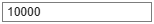0.5/100; % Power threshold (%), compared to 1st path, determining end of clusters [pathArrivalTimes, pathAveragePowers, pathPhases] = helperPathModeling(env, clusterArrivalTimes, clusterEnergies, Tend, cfgBPRF.SampleRate);```

• Nakagami parameters: Now that the average power has been determined for each path, the next step is to enable small-scale fading by determining the different channel realizations according to a Nakagami distribution. The channel model recommends that the shape parameter `m` of the Nakagami distribution is a lognormal random variable, whose mean and variance are dependent on the environment and the path delay.

`nakagamiM = helperNakagamiParameters(env, pathArrivalTimes);`
• Nakagami random variables: You can obtain a Nakagami random variable with shape parameter `m` and spread parameter `Ω` (the average path gain) from the square root of a gamma-distributed random variable that has shape parameter `k = m` and scale parameter `θ = Ω/m` . This example generates a gamma-distributed random variable with a sequence of steps, including the Ahrens-Dieter method . The Nakagami random variables are created within the `helperSingleChannelRealization` function.

• Simulation: Small-scale (Nakagami) fading is applied throughout the input signal. The timescale of fading is determined by the following 2 inputs:

```sampleDensity =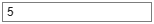100000; maxDopplerShift =5; % in Hz```

New path gains are generated with a rate equal to `maxDopplerShift x 2 x sampleDensity`. All the channel realizations occur within `helperAllChannelRealizations`, and its `pathGains` output contains all channel realizations.

```[rxSignal, pathGains] = helperApplyChannel(rxSignal, clusterArrivalTimes, ... pathArrivalTimes, pathAveragePowers, pathPhases, nakagamiM, cfgBPRF.SampleRate, sampleDensity, maxDopplerShift); helperVisualizeChannelGains(clusterArrivalTimes, pathArrivalTimes, pathGains);```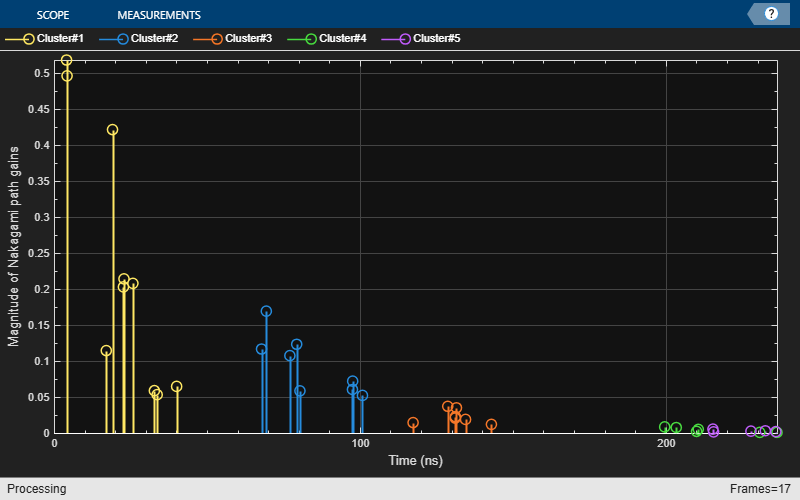Comparing the scatter plots of the UWB channel input and output demonstrates the effects of the multipath channel.

`scatterplot(waveBPRF);`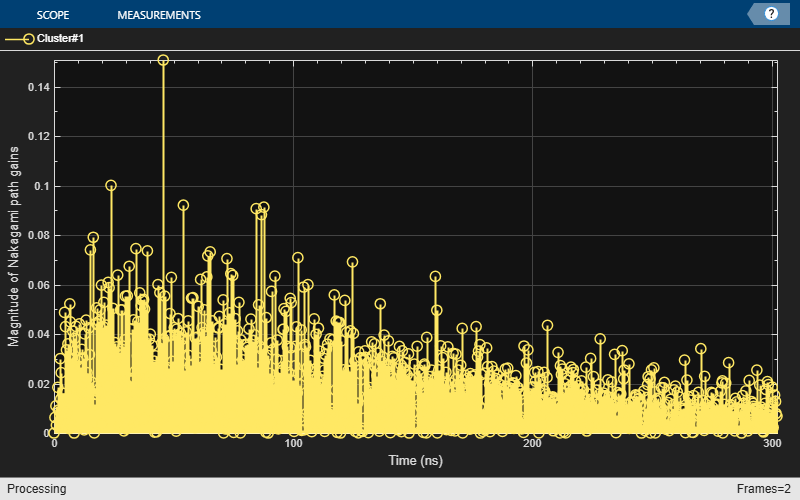The IEEE 802.15.4a/z signal contains ternary symbols at -1, 0, and 1. Other constellation points are the result of pulse (Butterworth) filtering and frequency-dependent path loss.

`scatterplot(rxSignal);`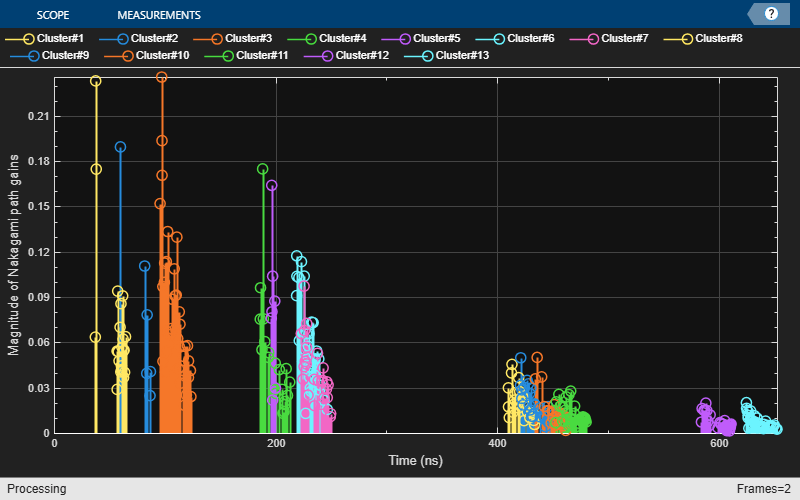The multipath channel introduces complex "noise" as a result of the complex path gains and inter-symbol interference.

### Environment Comparison

You can generate the channel gains for different environments, to illustrate key differences in their power delay profiles. Towards this end, use the `uwbChannel` System object™, which encapsulates all prior components of the UWB channel model, in a single utility.

• Alternate PDP: First, the industrial NLOS environment differs in that it consists of a single cluster with regular tap spacings. The PDP shape first increases and then decays.

Here, we use a variant of `uwbChannel` that only generates the path gains and does not filter the transmitted signal. The default behavior of `uwbChannel` is to filter the transmitted signal and output the received one.

```industrialUWBChannel = uwbChannel('Industrial', false, ... TransmitPower = 1, ... Distance = 10, ... ChannelNumber = 0, ... LastPathThreshold = 0.05, ... SampleRate = 499.2*10*1e6, ... SampleDensity = 1e5, ... MaxDopplerShift = 5, ... ChannelFiltering=false); % waveTx = lrwpanWaveformGenerator(psdu, cfgBPRF); % start with a clean signal % [waveRx, pathGains] = industrialUWBChannel(waveTx); % pass Tx signal through the wireless channel, with ChannelFiltering = true (default) pathGains = industrialUWBChannel(); % only obtain channel realization s = info(industrialUWBChannel); helperVisualizeChannelGains(s.ClusterArrivalTimes, s.PathArrivalTimes, pathGains);```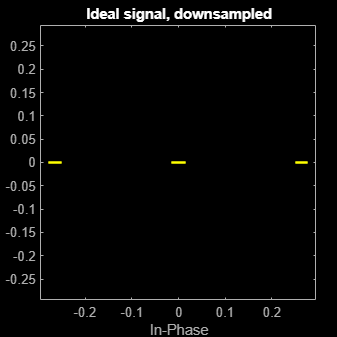• Outdoor environments: The outdoor UWB environment differs in that its average number of clusters is typically substantially higher.

```outdoorUWBChannel = uwbChannel('Outdoor', false, ChannelFiltering=false); pathGains = outdoorUWBChannel(); % only obtain channel realization s = info(outdoorUWBChannel); helperVisualizeChannelGains(s.ClusterArrivalTimes, s.PathArrivalTimes, pathGains);```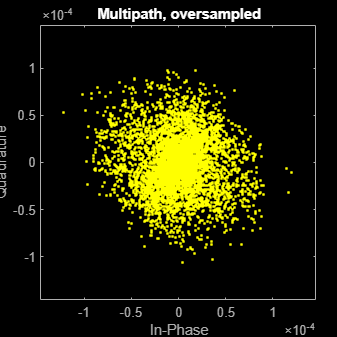### Further Exploration

To further explore this example, you can modify the environment type and the LOS setting. You can give different values for the transmitted power, the distance between the two link endpoints, the channel number, and the ratio threshold comparing the powers of the first and the last path within a cluster.

You can also explore the implementation of the channel configuration and channel filtering objects as well as the helper functions that implement different components of the UWB channel model:

### Bibliography

1. A. F. Molisch et al., “IEEE 802.15.4a Channel Model-Final Report,” Tech. Rep., Document IEEE 802.1504-0062-02-004a, 2005.

2. "IEEE Standard for Low-Rate Wireless Networks," in IEEE Std 802.15.4-2020 (Revision of IEEE Std 802.15.4-2015), pp.1–800, 23 July 2020, DOI: 10.1109/IEEESTD.2020.9144691.

3. "IEEE Standard for Low-Rate Wireless Networks--Amendment 1: Enhanced Ultra Wideband (UWB) Physical Layers (PHYs) and Associated Ranging Techniques," in IEEE Std 802.15.4z-2020 (Amendment to IEEE Std 802.15.4-2020), pp.1–174, 25 August 2020, DOI: 10.1109/IEEESTD.2020.9179124.

4. A. Saleh and R. A. Valenzuela, “A statistical model for indoor multipath propagation,” IEEE J. Selected Areas Comm., Vol. 5, pp. 138–137, February 1987.

5. https://en.wikipedia.org/wiki/Nakagami_distribution#Generation

6. https://en.wikipedia.org/wiki/Gamma_distribution#Generating_gamma-distributed_random_variables